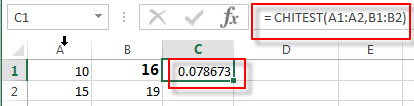# Excel CHITEST Function

This post will guide you how to use the CHITEST function with syntax and examples in Microsoft excel.

### Description

The Excel CHITEST function returns the value from the chi-squared distribution for the statistic and the appropriate degrees of freedom. So you can the chi-square test to calculate the probability that the differences between two given date sets in Excel.

The CHITEST function is a build-in function in Microsoft Excel and it is categorized as a Statistical Function.

The CHITEST function is available in Excel 2016, Excel 2013, Excel 2010, Excel 2007, Excel 2003, Excel XP, Excel 2000, Excel 2011 for Mac.

### Syntax

The syntax of the CHITEST function is as below:

`= CHITEST(actual_range, expected_range)`

Where the CHITEST function arguments are:

• Actual_range -This is a required argument. The actual range of data to test against expected range.
• expected_range – This is a required argument.  The range of data that contains the ratio of the product of row totals and column totals to the grand total.

Note:

• If actual_range and expected_range have a different number of data points, CHITEST returns the #N/A error value.

### Excel CHITEST Function Examples

The below examples will show you how to use Excel CHITEST Function to get the value from the chi-squared distribution.

1# to calculate the right-tailed probability of the chi-square distribution, using the following formula:

`= CHITEST(A1:A2,B1:B2)`### Related Functions

• Excel CHIDIST function
The Excel CHIDIST function returns the right-tailed probability of the chi-squared distribution.The syntax of the CHIDIST function is as below:= CHIDIST (x,deg_freedom).…
• Excel CHIINV function
The Excel CHIINV function returns the inverse of the right-tailed probability of the chi-squared distribution.  For example, if the probability is equal to CHIDIST(x,…), then the CHIINV(probability,…) is equal to x.The syntax of the CHIINV function is as below:= CHIINV (probability, deg_freedom).…